Instabilities in stratified mixing layers

Gelfgat & Kit, JFM, 2006, vol. 552, pp. 189-227

Temporal formulation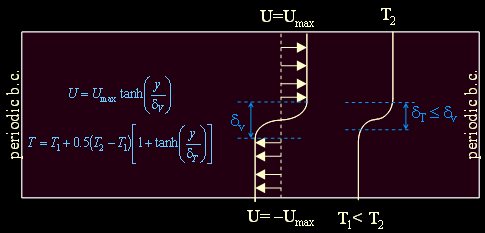Perturbation: u’,v’,p’,T’ ~ exp(iax)

Governing parameters:   Re,  Ri,  Pe, R=dv/dT

Calculation of 2D case:      animation

Calculation of 3D case:     animation1    animation2

Spatial  formulation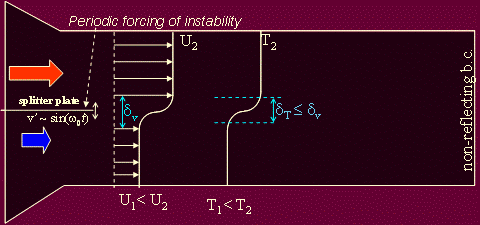Spatial wavenumber of excitation: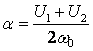Spatial wavenumber of perturbation: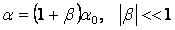Length scale: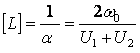Governing parameters:   Re,  Ri,  Pe,  l, , R=dv/dT

Calculation of 2D case:    animation

Scales and governing parameters:

 Spatial problem Temporal problem Perturbation: ~exp(iw0t) (forced) ~exp(iax) Velocity ratio, l l=(U2 – U1) / (U2 + U1) L, length (U2+U1) / 2w0 a-1 t, time L / (U2–U1) =1/ 2lw0 L /Umax =1 / 2aUmax V, velocity U2 – U1 2Umax p, pressure r(U2–U1)2 4rU2max T, temperature T2 – T1 T2 – T1 Governing parameters: Re = VL/n (U2–U1) (U2+U1) / 2w0n 2Umax / an Ri = gbDTL/V2 gb(T2 – T1) / 2w0l(U2–U1) gb(T2 – T1) / 4aU2max Pe = VL/c (U2–U1) (U2+U1) / 2w0c 2Umax / ac

General form of perturbation:       A(y)exp[i(ax+azz+wt)]

Criterion for the temporal instability:   a=a0, Im(w)<0

Criterion for the spatial instability:       w=w0, Im(a)<0

or   Im(b)<0

to solve for both temporal and spatial instabilities

Possibility for 3D initial growth